# MP Board Class 12th Physics Important Questions Chapter 2 Electrostatic Potential and Capacitance

## MP Board Class 12th Physics Important Questions Chapter 2 Electrostatic Potential and Capacitance

Electrostatic Potential and Capacitance Important Questions Objective Type Questions

Question 1.
Choose the correct answer of the following:

Question 1.
The SI unit of electrical capacitance:
(c) Coulomb
(d) Stat coulomb.

Question 2.
The potential difference between the plates of a capacitor is constant. A dielectric medium is filled instead of air in between the plates. The intensity of electric field will:
(a) Decrease
(b) Remains unchanged
(c) Become zero
(d) Increase.
(b) Remains unchanged

Question 3.
On replacing the air by an insulating material between the plates of a capacitor its capacity:
(a) Remains unchanged
(b) Increases
(c) Decreases
(d) Nothing can be said.
(b) Increases

Question 4.
On increasing the separation between the plates of a parallel plate capacitor its capacitance :
(a) Remains unchanged
(b) Increases
(c) Decreases
(d) Nothing can be said.
(c) Decreases

Question 5.
When two capacitors are joined in series each capacitor will have the same :
(a) Charge
(b) Potential
(c) Charge and potential
(d) Neither charge nor potential.
(a) ChargeQuestion 6.
When two capacitors are joined in parallel each capacitor will have the same:
(a) Charge and potential
(b) Only charge
(c) Only potential
(d) Neither charge nor potential.
(c) Only potential

Question 7.
Two capacitors of equal capacitance first connected in parallel then connected in series. What is the ratio of their capacities in both the cases:
(a) 2 : 1
(b) 1 : 2
(c) 4 : 1
(d) 1 : 4.
(c) 4 : 1

Question 8.
The formula of capacitance of a spherical conductor is:
(a) C = $$\frac { 1 }{ 4π{ £ }_{ 0 }R }$$
(b) C = 4πt£0R
(c) C = 4πr£0R2
(d) C = 4π£0R3
(b) C = 4πt£0R

Question 2.
Fill in the blanks:

1. 1 farad = one coulomb/ ……………
2. 1 farad = …………… stat farad.
3. Dimensional formula of capacitance is ……………
4. is a device in which with or out changing in shape or size of a conductor its capacitance can be increased ……………
5. On increasing the distance between the plater of a parallel plate capacitor its capacity ……………
6. Three capacitor each of 3pF are joined in series their equivalent capacitance will be ……………
7. The dimensional formula of electric potential is ……………
8. The potential due to a point charge q at a distance r is given as ……………
9. The potential difference = Intensity of electric field × ……………
10. The increase in kinetic energy of a charge q when it is accelerated by a potential difference V is ……………
11. Due to presence of dielectric medium the potential ……………
12. The work done in moving a charge perpendicular to the electric field is ……………
13. The potential of earth is considered to be ……………

1. 1 Volt
2. 9 × 1011
3. [M-1L-2T4A2]
4. Capacitor
5.  Decreases
6. lµF
7. [ML2T-3 A-11]
8. V = $$\frac { 1 }{ 4π{ £ }_{ 0 }R }$$ $$\frac { q }{ r}$$
9. Distance between the two point
10. qV
11. Decreases
12. Zero
13. Zero.Question 3.
Match the Column: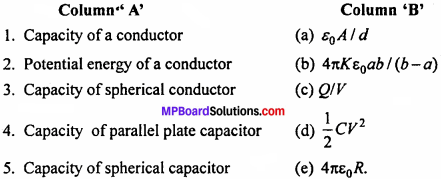1. (c) Q/V
2. (d) $$\frac { 1}{2}$$ C/V2
3. (e) 4π£0R
4. (a) £0A/d
5. (b) 4π£0ab / (b – a)

Question 4.
Write the answer in one word / sentence:

1. What is the potential of earth. Write SI units ?
2. What will be the electric field intensity inside a hallow sphere ?
3. In which direction of electric dipole, electric potential is zero ?
4. What is the net charge of a charge condenser ?
5. What quantity remains constant when the condenser are connected in series ?
6. What quantity remain constant when the conductor are connected in parallel ?

1. Zero, volt
2. Zero
4. Zero
5. Charge
6. Potential difference.Electrostatic Potential and Capacitance Important Questions Very Short Answer Type Questions

Question 1.
What do you understand by equal potential surface ?
The surface of the conductor where potential is in every point is called equal potential surface.

Question 2.
Write the name of the physical quantity whose SI unit in J/C. Is it a scalar or vector ?
Electric potential, it is a vector quantity.

Question 3.
Draw a equi potential surface for a unit charge.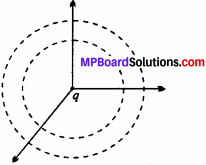Question 4.
If the potential of a conductor increases by one volt when one coulomb of charges is given to it, then the capacity of the conductor is said to be one farad.

Question 5.
On going in direction of electric lines of force, electric potential decreases or increases.
Electric potential decreases.

Question 6.
Give an example in which electric field is non-zero but potential is zero.
At broad-side-on position of an electric dipole electric field is non-zero and potential is zero.Question 7.
Does electron try to go toward high potential area or low potential area ?
Since electron is negatively charge so it tries to go toward high potential area.

Question 8.
Potential between two parallel surface are same. The distance between them is R. If a charge q is bought from one surface to another, then what will be the work I done to do this ?
Amount of work done will be zero on both the surface are equipotential.

Question 9.
If area of a plate of a parallel plate condenser in made half. Will it behave as condenser.
When area of the plate if a parallel plate condenser is made half. Its capacity become half. Therefore it will not act as condenser.

Question 10.
A capacitor of capacity C is charged with potential difference V. What will be the magnitude of electric flux passing through the surface of it ?
Zero.

Question 11.
Why condenser are used in computer’s ?
Condenser are used as memory chip in computer.

Question 12.
Write one use of capacitor ?
To accumulate electric charge.Electrostatic Potential and Capacitance Important Questions Short Answer Type Questions

Question 1.
What is potential ? Is it a vector or scalar quantity ?
Work done in bringing a unit positive charge from infinity to a point in the I electric field is called potential at that point. If charge q is brought from infinity to a point and IT work is done.
∴ V = $$\frac {W}{p}$$
It is a scalar quantity.

Question 2.
Can same amount of charge be given 1 and a solid sphere of same radii, if they have same potential ?
No, because capacities of both spheres of same radii are always equal. Therefore i both the spheres can hold same amount of charge at same potential.

Question 3.
What is meant by capacity of a conductor ? Give its unit.
The capacity of a conductor is defined by the charge given to the conductor, which increases its potential through unity.
Capacity = $$\frac {Charge}{Potential}$$
or C = $$\frac {q}{v}$$
Its SI unit is farad.

Question 4.
The surface of any conductor is always equipotential. Why ?
Or
The potential at every point on a charged conductor is same. Why ?
All the points of the surface of a conductor are in electrical contact with one another. If the potential is not equal then the charges will flow from higher potential to lower potential till the potential of both the points on the surface becomes same. This will give rise to electrodynamics situations. Thus, the surface of a conductor is always equipotential.Question 5.
What would be the work done if a point charge +q is taken from a point A to point B on the circumference of a circle with another point charge +q at the center:
The points A and B are at same distance from the charge + q at the center, so VA = VB So, work done, W= q0 (VA – VB) = 0.Question 6.
Explain the meaning of capacity of a capacitor. What will be the effect on capacity of a parallel plate capacitor if a dielectric medium of dielectric constant k is filled in between the plates ?
The capacity of a capacitor, is equal to charge given to one of its plates which produces unit potential difference across the plates. In this case capacity increases, it becomes k times its initial value.

Question 7.
What will be the change in the value of charge and potential difference between the plates of a parallel plate capacitor, if after charging its battery is removed and distance between its plates is reduced ?
Charge remains same but potential difference decreases.

Question 8.
Two equipotential surface does not intersect each other, why ?
Electric lines of forces are always perpendicular to equipotential surface. If two equipotential surface intersect each other then at the point of intersection there will be two direction of electric fields which is impossible. Therefore they does not intersect each other.

Question 9.
Why must electrostatic field be normal to the surface at every point of a charged conductor ?
If electric field is randomly directed, then it can be resolved, into two components. The horizontal component on this surface is E sin θ.
For electrostatic situation
£ sin θ = 0
⇒ sin θ = 0
⇒ θ = 0°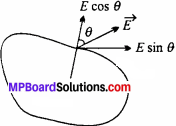So, the electric field is normal to surface.

Question 10.
The potential at any point inside the hollow conductor remains same. Why ?
When charge is given to a hollow conductor then the distribution of charge takes place on its upper surface. Therefore the intensity of electric field inside the conductor is zero. Hence, no work is done in moving unit positive charge inside it. Therefore potential at every point inside the conductor remains same.Question 11.
Can the potential be zero where electric field is not zero ?
Yes, the electric field on the equatorial line of a dipole is not zero but potential is zero.

Question 12.
What will be the effect on electric field, potential, difference, electric capacity and energy if a dielectric of dielectric constant K is filled between the plates of a capacitor ?
The electric field will become $$\frac {1 }{ K }$$times, potential difference will become $$\frac {1 }{ K }$$ times, electric field will become K time and energy will become $$\frac {1 }{ K }$$ times.

Question 13.
Can 1 coulomb charge be given to a sphere of radius 1cm ?
As we know that the formula of potential ¡s V = $$\frac { 1 }{ 4π { £ }_{ 0 } }$$ $$\frac { q }{ r }$$ …(1)
Given,q = lC, r = lcm = 10-2m
Putting these values in eqn. (1)
V = 9 × 109 × $$\frac{1}{10^{-2}}$$ = 9 x 1011volt
Where = $$\frac{1}{4 \pi \varepsilon_{0}}$$ =  9 × 109 in SI unit.
This value of potential is greater than barrier potential of air. Therefore IC charge cannot be given to a sphere of radius 1cm.

Question 14.
In the shown figure what will be the work done to bring a z point charge from the point X to Y to Z ?
There the point Z and Y are situated on same equaipotential surface. Therefore work done to bring a point charge from A’to Zand from X to Z will be same.
.’. Wy= Wz.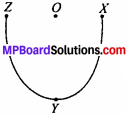Question 15.
Derive an expression for electric potential due to a point charge. Is it scalar or vector and why ?
Consider a point charge q placed at origin O. Potential at P has to be found out. Let the medium between charge ‘q’ and P has dielectric constant Er.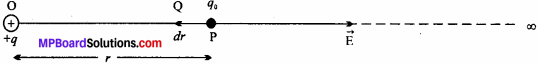Electric field at P due to charge q is
E = $$\frac{1}{4 \pi \varepsilon_{0} \varepsilon_{r}} \cdot \frac{q}{r^{2}}$$
The electric field $$\vec{E}$$ points away from the charge q. A force $$\vec{F}$$ = -q0 $$\vec{E}$$ has to be applied on the charge so that it can be brought near to q. The small work required to move the test charge q0 from P to Q through a small distance dr is given by dW = Fdr
= -q0 Edr
The total work done in moving the charge q0 from infinity to point P will be obtained by integrating the above equation as –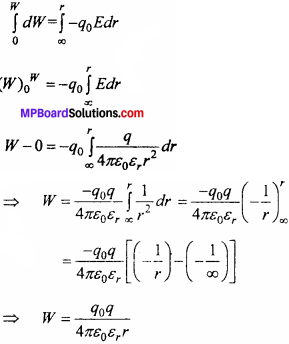But electric potential is defined as work done per unit test charge.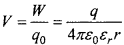Potential at P is V = $$\frac{1}{4 \pi \varepsilon_{0} \varepsilon_{r}} \cdot \frac{q}{r}$$
If medium between q and q0 is vacuum then £r = 1
Then , V =$$\frac{1}{4 \pi \varepsilon_{0}} \frac{q}{r}$$
This is the required expression.Question 16.
What are the factors affecting the potential of a charged
The factors affecting the potential of a charged conductor are:
1. Amount of charge on conductor:
By the formula V = $$\frac{1}{4 \pi \varepsilon_{0}} \frac{q}{r}$$ it is clear that
V ∝ q, hence more is the charge, more will be the potential of charged conductor.

2. Shape of conductor (Area of conductor):
If the charge is kept constant on a conductor and its surface area is decreased then the potential of conductor increases whereas on increasing the surface area its potential decreases. So the potential of a conductor is inversely proportional to the radius.

3. Presence of other conductor near the charged conductor:
If an uncharged conductor is brought near a charged conductor then the potential of the charged conductor decreases.

4. Medium surrounding the conductor:
Due to presence of insulating medium near the charged conductor its potential will decrease.

Question 17.
Define equipotential surface. Write its properties.
Equipotential surface:
An equipotential surface is the locus of all those points at which the potential due to distribution of charge remains same.

Properties:

• Potentials on every point are equal
• No work is done in moving a positive charge from one point to another
• The electrical lines of force are normal to the equipotential surface
• Two equipotential surfaces do not intersect each other.
• All the points on the surface of a conductor are in electric contact. If the potentials are not same then the
• charge will flow from higher potential to lower potential till the potential of both the points become same.
• Thus the surface of a conductor is always equipotential.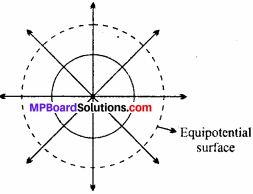Question 18.
Obtain a relation between electric Held intensity and potential difference.
Or
Prove that E = $$\frac { dv }{ dr }$$where symbols have their usual meanings.
Suppose A and B are two points in the electric field of charge q. The direction of electric field is radially outwards from A to B. Suppose the distance between A and B is very small (i. e., dr) then the electrie field between A and B can be taken as uniform. As the potential is inversely proportional to distance hence potential at A is more than that of B. Let the potential at B is V then that at A is V + dV.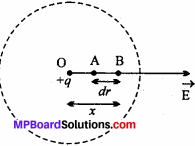Work done in bringing the test charge q0 from B to A is –
dW = q0dV …….(1)
Force acting on q0 will be
F = q0 E
Work done in bringing the test charge against the repulsion force will be
dW = -q0Edr
(Work = Force x Displacement) ………(2)
The negative sign shows that the direction of displacement and direction of force are opposite to each other.
From eqns. (1) and (2), we get,
q0dV = q0Edr
or dV = -Edr
E = – $$\frac { dv }{ dr }$$
This is required relation between intensity of electric field and potential difference.

Question 19.
Prove that capacity of an isolated spherical conductor is directly proportional to its radius.
Or
Derive an expression for the capacity of a spherical conductor.
The capacity of a conductor is its ability to store electrical energy and it is equal
to that charge which increases its potential by unity.
∴ Capacity = $$\frac { Charge }{ Potential }$$Capacity of an isolated spherical conductor:
Let us consider about a spherical conductor of radius r. The charge + Q is given to it. The charge will be distributed on its surface uni- formly. Therefore the lines of force will be emitted normally to the surface seem to becoming from its center. Hence, we can suppose that all the charges are kept at the centre.
∴ Potential on the surface V = $$\frac{1}{4 \pi \varepsilon_{0}} \frac{Q}{r}$$
But capacity C = $$\frac { Q }{ V }$$
Putting the value of V, we get
C = $$\frac{Q}{\frac{1}{4 \pi \varepsilon_{0}} \frac{Q}{r}}$$
C = 4πE0r
C ∝ r.
Thus, the capacity is proportional to the radius of the spherical conductor.Question 20.
What do you mean by a capacitor ? Explain its principle.
The capacitor is a device by which the capacitance of a conductor is increased without changing its size or volume. Actually it stores electrical energy.
Principle of capacitor:
Let A be a charged conducting plate. Another uncharged conductor plate B is brought near to A, therefore due to induction negative charges will be induced on the front surface and positive charges on the other side of plate B.
Now, the negative charge reduces the potential while the positive charge increases.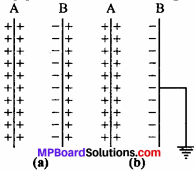As the negative charge is nearer therefore the potential of plate A decreases. Now, the plate B is earthed then the free positive charge will go to earth and hence the potential of A decreases by more value.
C = $$\frac { Q }{V}$$
As V decreases, C will increase. This arrangement is called capacitor or condenser.

Question 21.
Derive an expression for parallel plate capacitor.
Let A and B be two plates of a parallel plate capacitor separated by a distance d apart. Area of each plate is A and dielectric constant of the medium between them is Er Now, plate A is given + Q charge. Therefore, – Q charge will be induced on the nearer surface of the plate B and + Q charge on the other side. As B is connected to earth, + Q charge of B will go to earth. Let the charge density of A is cr, therefore that of B will be -σ.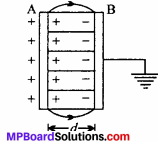Now, σ = $$\frac { Q }{A}$$
Intensity between the plates will be given by
E = $$\frac{\sigma}{\varepsilon_{0} \varepsilon_{\mathrm{r}}}$$
E = $$\frac{Q}{A \varepsilon_{0} \varepsilon_{r}}$$
But, potential difference between the plates A and B is
V = Electric field intensity ×
Distance between to plates = Ed
V = $$\frac{Q}{A \varepsilon_{0} \varepsilon_{s}} \cdot d$$
But, C = $$\frac{Q}{V}=\frac{Q}{\frac{Q d}{A \varepsilon_{0} \varepsilon_{r}}}$$
C = $$\frac{\varepsilon_{r} \varepsilon_{0} A}{d}$$
This is the required relation.
For air or vacuum, Er = 1
C = $$\frac{\varepsilon_{0} A}{d}$$

Question 22.
Three capacitors of capacitance’s C1 C2 and C3 are connected in series. Derive an expression for the equivalent capacitance.
The given figure shows three capacitors of capacitances C1 C2 and C3 con – nected in series. A potential difference of V is applied across the combination, charges of + Q and – Q are developed on the plates of the capacitor.
Potential difference across the individual capacitors will be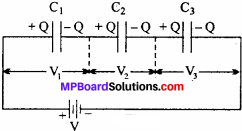V1 = $$\frac{Q}{C_{1}}$$ , V2 = $$\frac{Q}{C_{2}}$$, V3 = $$\frac{Q}{C_{3}}$$ …….(1)
The sum of these must be equal to the applied potential difference V.
V = V1 + V2 + V3 ………(2)
Let C be the equivalent capacitance of the series combination
∴ C = $$\frac { Q }{ V }$$ or V = $$\frac { Q }{ C }$$ ………..(3)
V1 + V2 + V3 = $$\frac { Q }{ C }$$ [from equ..(2)]
$$\frac{Q}{C_{1}}$$ + $$\frac{Q}{C_{2}}$$ + $$\frac{Q}{C_{3}}$$ = $$\frac{Q}{C}$$ [from equ..(1)]
Q($$\frac{1}{C_{1}}$$ + $$\frac{1}{C_{2}}$$ + $$\frac{1}{C_{3}}$$) = $$\frac{Q}{C}$$
$$\frac { 1 }{ c }$$ = $$\frac{1}{C_{1}}$$ + $$\frac{1}{C_{2}}$$ + $$\frac{1}{C_{3}}$$
This is the required expression.Question 23.
Three capacitors of capacitance’s C1 C2 and C3 are connected in parallel. Derive an expression for the equivalent capacitance C.
Consider three capacitor of capacitance’s C1 C2 and C3 connected in parallel. A potential difference V is applied across the combination. Charges set up in the individual capacitor will be.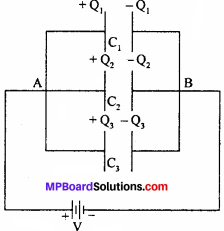Q1 = C1V, Q2 = C2V,Q3 = C3V …(1)
Total charge stored in the parallel combination is
Q = Q1 + Q2 + Q3 ……….(2)
If C is the equivalent capacitance of the combination
Then, C = $$\frac { Q }{ V }$$ Q = CV …………(3)
Q1 + Q2 + Q3 = CV [from eq. (2)]
C1V + C2V + C3V = CV [from eq. (1)]
V(C1 + C2 + C3) = CV
C = C1 + C2 + C3
This is the required expression.

Question 24.
Derive an expression for the energy of a charged conductor.
Or
Prove that energy of a charge conductor is directly proportional to its square of potential.
The work done in charging a conductor is stored as energy in it. This energy is called electrostatic potential of conductor.

Formula derivation:
Let us consider about a conductor of capacity C which is given charge +Q due to which its potential becomes V. As the charge increases work done also increases. Let at any instant the potential of conductor be V due to charge q.
∴ C = $$\frac { q }{ v}$$
or v = $$\frac { q }{ C}$$
Now, at potential Kthe work done in giving the charge dq will be dW
∴ dw = Vdq
or dw = $$\frac { q }{ C}$$dq
Work done in charging the conductor from 0 to Q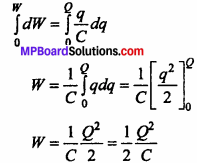This work done is stored as potential energy on the conductor. Energy of a charge conductor
U = $$\frac{1}{2} \frac{Q^{2}}{C}$$
But Q = CV
∴ U = $$\frac{1}{2} \frac{C^{2} V^{2}}{C}$$
or U = $$\frac{1}{2} C V^{2}$$
∴ U ∝ V2 because C is constantQuestion 25.
Prove that on connecting two charged conductors, charges distribute on them according to their capacities.
When two isolated charged conductors A and B are connected by a thin wire, charge flows from the conductors at high potential to the conductor at low potential till the potential of both A and B became equal. The phenomenon involved is called distribution of charges and the total charge of the entire system remains conserved. Let the capacitance of A and B be C1 and C2, the charges be Q1 and Q2 respectively. Then the potentials are V1 and V2 respectively.
∴ Initially, Q1 = C1V1 and Q2 = C2V2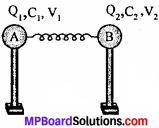The conductors are joined by a wire of negligible capacitance, the charges flow from- the conductor at higher potential to the conductor at lower potential till the potentials on each conductor become equal.
The net charge on the system,
Q = Q1 + Q2
The common potential, V = $$\frac { Total charge }{ Total capacitance }$$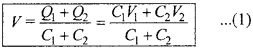After the potential becomes equal let the charge on A 1 be Q1 and charge on A2 be Q2.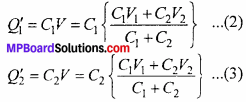Dividing eqn. (2) by eqn. (3), we get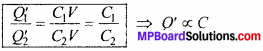When the conductors are joined, then the charges get distributed in the ratio of their capacities.

Question 26.
Obtain an expression for potential due to a group of point charges.
Or
Derive the expression for potential energy.
Consider a group of point charges q1,q2,q3……..qn which are situated at a dis-tance of r1, r2, r3…….. nn respectively from the point P. The potential due to these point charges is to be obtained at P. Now potential at P due to q1 is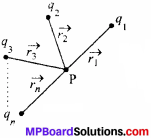potential due to q2,q3, ……… qnetc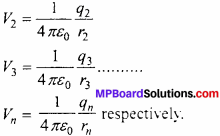Total potential at P will be V = V1 + V2 + V3 + ……….. + Vn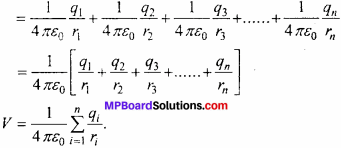This is the required expression.

Electrostatic Potential and Capacitance Important Questions Long Answer Type Questions

Question 1.
Derive the expression for the capacity of a parallel plate capacitor, when the medium between the plates is partially filled by a dielectric medium.
Let A and B are parallel plates of a capacitor. The distance between the plates is d and plate of thickness t and dielectric constant Er is introduced.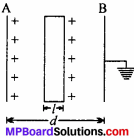Now, plate A is given charge +Q.
Let the charge density be σ.
∴ σ = $$\frac { Q}{ A}$$
Intensity of field in air ,
E_{0}=$$\frac{\sigma}{\varepsilon_{0}}=\frac{Q}{\varepsilon_{0} A}$$
If the intensity of field inside the dielectric medium be E, then
Dielectric constant = $$\frac {Electric field in vacuum}{ Electric field in medium}$$
or $$\varepsilon_{r}=\frac{E_{0}}{E}$$
or E = $$\frac{E_{0}}{\varepsilon_{r}}=\frac{Q}{\varepsilon_{0} \varepsilon_{r} A}$$
Now, potential difference between A and B,
V=E0 (d – t) + Et, [(d – t) is vacuum distance]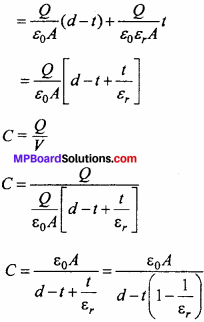This is the required expression.
Metal is a conductor. When metal is used in place of the dielectric, it will conduct electricity and the potential difference will become zero. So, capacitor will not work.

Question 2.
Calculate the loss of energy, when two charged conductors are connected.
Or
The capacities of two conductors are C1 and C2, Q1 and Q2 charges are given to them so that their potentials become V1 and V2 respectively. If they are connected by a wire, then calculate the following:

• Common potential
• Loss of energy.

or
prove that when two charged conductors are connected, there will be a loss of energy
Or
In redistribution of charges, is there a loss of energy ? Deduce an expression to confirm the answer.
Let A and B be two conductors of capacities C1 and C2 respectively. When charges Q1 and Q2 are given separately the potentials become V1 and V2 respectively.Total charges, Q = Q1 + Q2 ………..(1)
But, Q1 = C1V1 and Q2 = C2V2
By eqn. (1), we get
Q = C1V1 + C2V2
Total capacity, C = C1 + C2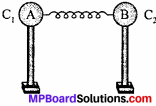(1) Common potential:
Let the conductors are connected by a wire and the common potential becomes-V.
Q1 + Q2 = (C1 + C2)V
V = $$\frac{Q_{1}+Q_{2}}{C_{1} + C_{2}}$$
V = $$\frac{C_{1} V_{1}+C_{2} V_{2}}{C_{1}+C_{2}}$$ ……..(2)
This is the expression for the common potential.

(2) Loss of energy: Total energy of the conductors before connection: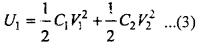and total energy after connection,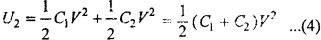Putting the value of V from eqn. (2) in eqn. (4), we get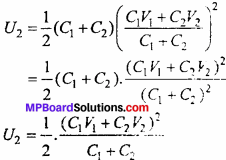Hence, difference of energy’,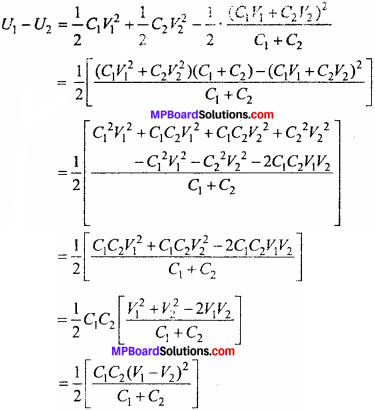(V1 – V2)2 is positive, hence (V1 – V2) is positive. Hence, during redistribution, there will be always loss of energy.
i.e., U1 – U2>0 ⇒ U1>U2
i.e., energy before joining is greater than energy after joining.
The loss in energy,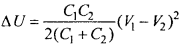Question 3.
Explain the construction and working of Van de Graaff generator. Write its uses.
Van de Graaff generator is a machine which produces electricity of about 107 V or more potential difference.
Construction:
It consists of a large metallic sphere S of diameter 5 m, mounted on high insulating support PP about 15 m high. An endless insulating belt made up of rubber passes over the pulleys p1 and P2. A motor rotates p1 C1 and C2 are two metallic combs called spray comb and collecting comb respectively. C1 is connected to S. To prevent the leakage of charge, the generator is put inside a large enclosure filled with gas at 15 atm. pressure. This iron enclosure is connected to earth.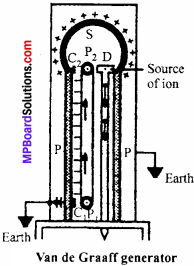Working:
The comb C1 is connected to the positive terminal of the battery, therefore the surface density of the points becomes very high which causes the wind present nearby it to get charged. Thus, the spray comb sprays the charge on the belt. Now, the electric wind moves up to the collector comb C2 When it reaches in front of the collector comb C2 opposite charge induces on the tip to neutralize the same type of charge. The negative charge wind of C2, cancels the positive charge of the belt. Thus, by the repeated actions more and more positive charge is induced on sphere, hence its potential increases to about 107volts or more.

Uses:

• To generate high potential.
• To accelerate the positive particles such as protons, Deuteronomy, are particle etc. and used in nuclear disintegration.Question 4.
When Anil opened the cap of the tap, then he found no water in coming out of it. Then he opened the cap of the water tank and found no water in the tank. To fill up water in the water tank he switch on the switch of the motor and found motor is not starting. Then he called the electric technician. The technician said him on checking that the condenser of the motor is not functioning. On replacing capacitor, the motor start working.

Answer the following questions:

1. What values does Anil exhibits ?
2. What is the function of condenser ?
3. What is total charge on a charged condenser ?
4. The capacity of a capacitor is 3pF. If it is charged up to 100 V potential difference, then what will be charged stored in it ?

1. Anil exhibited his presence of mind.
2. It accumulate charge and hence it conserved energy.
3. Net charge on a condenser is zero.
4. C = 3µF = 3 × l0-6 F, V = 100V

∴ By formula Q = CV = 3 × l0-6 × l00
or Q = 3 × 10-4C.

Electrostatic Potential and Capacitance Important Questions Numerical Questions

Question 1.
Can 1 coulomb charge be given to a sphere of radius 1cm ?
No.
As we know that the formula of potential is V = $$\frac{1}{4 \pi \varepsilon_{0}} \frac{q}{r}$$ …(1)
Given, q = 1C, r = 1cm = 10-2m
Putting these values in eqn. (1)
V = 9 × 109 x $$\frac{1}{10^{-2}}$$ = 9 × 1011volt
Where = $$\frac{1}{4 \pi \varepsilon_{0}}$$ = 9 × 109 in SI unit.
This value of potential is greater than barrier potential of air. Therefore lC charge cannot be given to a sphere of radius 1cm.

Question 2.
You are given three capacitor of 4pF each. How they will be combined to obtain resultant capacity of 6pF ?
Solution:
Given : Q = C2 = C3 = 4µF
When two capacitor is joined in series and third capacitor joined parallel with them, then resultant capacity is obtained as 6µF.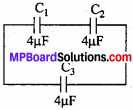C1 ,C2 is in series, its resultant (C’) is
$$\frac { 1 }{ c}$$ = $$\frac{1}{C_{1}}+\frac{1}{C_{2}}$$
or $$\frac { 1 }{ c}$$ = $$\frac { 1 }{ 4}$$ + $$\frac { 1 }{ 4}$$ = $$\frac { 2 }{ 4}$$
or C = $$\frac { 4 }{ 2}$$ = 2µF.
C and C3 is in parallel combination,
Its resultant C is C = C + C3
C = 2 + 4 or C = 6µF.Question 3.
A hollow metallic sphere of radius 0-1 m is given 6pC. Calculate its potential:

1. At the surface of sphere
2. At the center.

Solution
Given, r = 01 m, q = 6µC = 6 x 10-6 C
Formula: V = $$\frac{1}{4 \pi \varepsilon_{0}} \frac{q}{r}$$

1. Potential at the surface:
V = 9 × 109 × $$\frac{6 \times 10^{-6}}{0 \cdot 1}$$
V = 54 × 104
V- 5.4 × 105 volt.

2. At the center:
Inside the sphere the potential remains same and equal to that on the surface hence V = 5 . 4 x 105volt.

Question 4.
A test charge is moved from A to B, B to C and A to C in an electric field E as shown in the figure :Find (1) Potential difference between A and C
(2) At which point electric potential will be high and why ?
Solution:
1. In right angled ∆ABC
AB2 = AC2 – BC2 = 52 – 32
∴ AB = 4 = dr
BC is perpendicular to electric field, therefore potential will be same at B and C.
VA – VC = VA – VB = -Edr = -4E
2. Therefore potential at point C will be more than potential of point A.Question 5.
Identical water droplets, having equal charge on each are combined to form a big drop. Compare the capacity of bigger drop with that of a small drop.
Solution:
Let radius of small droplet = r
Radius of the big drop = R
Volume of big drop = Volume of 27 droplets
$$\frac { 4 }{ 3 }$$ πR3 = 27 × $$\frac { 4 }{ 3 }$$ πR3
or R3 = 27r3
or R3 = (3r)3
or R = 3r
or $$\frac { R }{ r }$$ = $$\frac { 3}{ 1 }$$
Since, C ∝ radius
or $$\frac{C_{1}}{C_{2}}=\frac{r_{1}}{r_{2}}$$
or $$\frac{C_{1}}{C_{2}}$$ = $$\frac { 3}{ 1}$$ = 3
or C1 = 3C2
The capacity of bigger drop is three times that of smaller one.

Question 6.
How three capacitor of 3pF each can be combined such that their resultant capacity is :

1. 9pF,
2. 4.5pF.

Solution:
1. When the three capacitor is joined in parallel, then
C = C1 + C2 + C3
= 3 + 3 + 3 = 9µF.

2. When two capacitor are joint in series, then resultant C’ is
$$\frac { 1 }{ c}$$ = $$\frac{1}{C_{1}}+\frac{1}{C_{2}}$$
= $$\frac { 1 }{ 3}$$ + $$\frac { 1 }{ 3}$$ = $$\frac { 2 }{ 3}$$
C = $$\frac { 3 }{2}$$ = 1.5 µF
Now C is joined in parallel with C3
C = C + C3 = 1.5 + 3 = 4.5µF.

Question 7.
The potential difference between two points is 10V. How much work is required to move a charge 100 pC from a point to the other ?
Solution:
Given, V= 10 volt, q = l00µC = l00 × l0-6C
Formula : w = qV
= 100 × 10-6 × 10
= 10-3 joule.Question 8.
Find the area of the plate of a 2F parallel plate capacitor, if the separation between the plates is 0.5 cm ?
Solution:
As C = $$\frac{\varepsilon_{0} A}{d}$$
A = $$\frac{C d}{\varepsilon_{0}}$$
Here, C = 2F, d= 0.5cm = 0.5 x 10-2m
A = $$\frac{2 \times 0 \cdot 5 \times 10^{-2}}{8.85 \times 10^{-12}}$$
= 1.13 × 109 m2 = 1130Km2

Question 9.
Two charges 5 x 10-8C and -3 x 10-8C are located 16 cm apart At what point, on the line joining the two charges, is the electric potential zero ? Take the potential at infinity to be zero. (NCERT)
Solution:
Case I.
Let electric potential be zero at point C lying at distance x from the positive charge.Given, q1 = 5 × 10-8 C;
q2 = -3 × l0-8 C
AC = x cm : CB = (16 – x) cm
Now, Potential at C is zero i. e.,
V1 + V2 = 0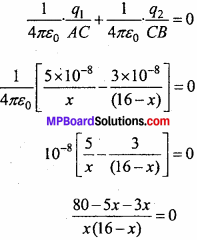-8x + 80 = 0
8x = 80
x = 19 cm
i.e., electric potential at a distance of 10 cm from positive charge will be zero.

Case II.
The other possibility is that the point C may also lie on produced AB.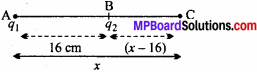Now, V1 + V2 = 0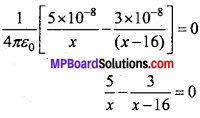5(x – 16) – 3x = 0
5x – 80 – 3x = 0
2x – 80 = 0
x = 40 cm from the positive charge

Question 10.
Determine the equivalent capacitance between A and B in the following circuits: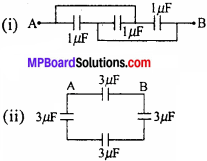Solution:
(i). Mark the junctions as C and D.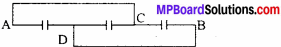(But C will be A and D will be B)
Draw the equivalent network, which is given below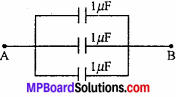Equivalent capacitance,
C = C1 + C2 + C3
or C = 1 + 1 + 1 = 3µF

(ii). To move from A to B, there are two paths P -1 and P – II.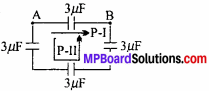(As A and B, the path is dislocated temporarily)
The capacitors in P -II are in series. So, the equivalent becomes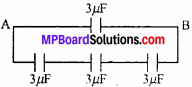The resultant capacity of series combination is
$$\frac { 1 }{ C’ }$$ = $$\frac { 1}{ 3 }$$ + $$\frac { 1 }{ 3}$$ + $$\frac { 1 }{3 }$$
= $$\frac { 3 }{ 3 }$$ = 1µF = C’ = 1µF
The equivalent further becomes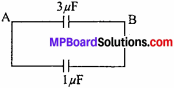Total capacity C = 3 +1 = 4µF.